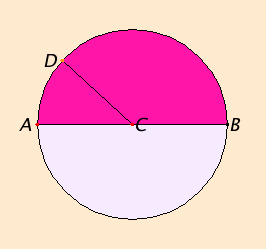# Definition 15

A circle is a plane figure contained by one line such that all the straight lines falling upon it from one point among those lying within the figure equal one another.

# Definition 16

And the point is called the center of the circle.

# Definition 17

A diameter of the circle is any straight line drawn through the center and terminated in both directions by the circumference of the circle, and such a straight line also bisects the circle.

# Definition 18

A semicircle is the figure contained by the diameter and the circumference cut off by it. And the center of the semicircle is the same as that of the circle.

## Guide

A definition such as this describes what circles are. Definitions do not guarantee the existence of the things they define. The existence of circles follows from a postulate, namely, Post.3.

Note that a circle for Euclid is a two-dimensional figure. But in modern mathematics, usually the word circle refers to what Euclid calls the circumference of a circle.

 The center of the circle in the diagram is the point C. It’s interesting that the English word “center” derives from the Greek word which also means a prod or a poker, and it refers to the fixed leg of a compass used to draw a circle. There is no assumption in the definition that there is only one center of a circle. Proposition III.1 gives a construction for the center of a circle, and the proof of that proposition shows that the center is unique. The (curved) line ABD that contains the circle is its circumference. Euclid typically names a circle by three points on its circumference. Perhaps a better translation than “circumference” would be “periphery” since that is the Greek word while “circumference” derives from the Latin. Euclid doesn’t have a term for radius other than “that from the center,” but radius is such a useful word that it is used here to translate “that from the center,” such as the radius CD.An example diameter is the line AB which passes through the center. Of course, a diameter is twice a radius, and since the radii are all equal to each other by definition, the diameters are also all equal to each other.

That diameters “also bisects the circle” should not be part of the definition, but either assumed as a postulate or proved as a proposition. It depends on the fact that circles are drawn on planes, and planes have constant curvature. The analogous figure on a surface of nonconstant curvature does not have this property. For such figures the two “semicircles” on either side of a “diameter” need not be equal.

Although circles are used throughout Book I to measure and transfer distances, the proper theory of circles doesn’t begin until Book III. That book begins with more definitions relating to circles including the equality of circles, when circles touch (are tangent to) lines and other circles, and so forth.